﻿ BER Performance of OFDM System in Noise and Fading Channel for Modified SL0 Sparse Algorithm
Journal of Telecommunications System & Management
• Research Article
• J Telecommun Syst Manage 2016, Vol 5(3): 141
• DOI: 10.4172/2167-0919.1000141

# BER Performance of OFDM System in Noise and Fading Channel for Modified SL0 Sparse Algorithm

Department of Electronics and Telecommunication Engineering, Terna Engineering College, Navi Mumbai, Maharashtra, India
*Corresponding Author: Umesh Mahind, Department of Electronics and Telecommunication Engineering, Terna Engineering College, Navi Mumbai, Maharashtra 400706, India, Tel: 22 61115444, Email: [email protected]

Received Date: Aug 31, 2016 / Accepted Date: Sep 15, 2016 / Published Date: Sep 30, 2016

### Abstract

In many communication systems, OFDM is extensively useful to reduce the bandwidth and for its enhancement ability of the data rate. In this paper, shows a comparison of the overall performance of OFDM system using different modulation schemes under the effect of AWGN channel. For OFDM signals, simulations are carried out with Rician fading as well as Rayleigh faded signal to recognize the effect of channel fading and to acquire optimum value of Bit Error rate (BER). In this paper, we proposed modified SL0 (mSL0) algorithm for sparse channel estimation in modern wireless communication system. We deal with simple OFDM system model for wireless communications. We address basic OFDM and related modulations, as well as techniques to improve the performance of existing SL0 algorithm for wireless communications. In this paper, the BER performance of OFDM-BPSK, QPSK and M-QAM system over generalized AWGN channel has been stated. This model is versatile enough to represent fading such Rician and Rayleigh. The flexibility of this model helps to analyse the severity of fading more deeply. We executed various simulations for OFDM signals to find out the best BER overall performance which are carried with generalized faded signal to understand the effect of channel fading.

Keywords: OFDM; Modern wireless channel; Modified SL0; AWGN, Rayleigh fading; Rician fading

#### Introduction

In wireless communication, OFDM (orthogonal frequency division multiplexing) has extensive application. In OFDM, each channel has profuse sub-channels having diverse frequencies which are also used in parallel transmission at low rate. Nowadays, it is further used in various wireless applications i.e., high performance wireless local area network (HYPERLAN), Digital Audio Broadcasting (DAB), Digital Video Broadcasting (DVB), Wi-MAX and Wi-Fi. OFDM is a special case of multi carrier transmission where a single data stream transmits over a number of lower rate subcarrier. It is worth citing here that OFDM can be work as a modulation technique or multiplexing technique. Along with MIMO technology, it will become promising technology for next generation wireless systems .

In order to recover the transmitted signal correctly, channel effect must be estimated and repaired at receiver. In this paper the performance evaluating parameter bit error rate, Eb/N0 and compressive sensing based sparse channel estimation methods are estimated and appropriate solution is recommended.

In this paper, section II is about Modern wireless communication channels. In the section III, OFDM system and sparse channel estimation is shown in mathematical form. In section IV, we proposed the modified SL0 (mSL0) algorithm for modern wireless communication channel. The last section is all about simulation. Performance of our proposed algorithm for modern wireless communication system is presented graphically. How it is affective for various modulation techniques is also shown in results.

#### Modern Wireless Communication System

In Figure 1, various modern wireless communication systems are shown. On the cellular devices which supports technologies like LTE, Wi-Max or Wi-Fi etc. These technologies promise to attain the higher data rates and are proven to be spectral efficient. Physical layer coding considers as a potential technology to improve the throughput of wireless systems. In wireless communications, the requirement of high speed transmission renewed interest for Mary modulation scheme, especially due to their potential to transmit more bits per trans-mitted symbol. 64-ary Quadrature Amplitude Modulation (64-QAM) is a well-known modulation technique used in wireless communications. Due to the high spectral efficiency, 64-QAM is an attractive technique for wireless communication. In wired communication, 256-QAM is employed, but it is not possible yet to use in wireless communication system, because of its complexity.

Practically, it is proved that MIMO technology as one means to improve performance for LTE as well as Wi-Fi with 2 × 2 solutions and technology is moving towards 4 × 4 MIMO. However, unlike other wireless technologies that have moved to 4x4 MIMO, like LTE, a significant design challenge has limited LTEs progress to 4 × 4 MIMO. Cellular systems are now evolving to utilize MIMO and the specifications for LTE-Advanced can support up to 8 × 8 MIMO, meaning eight downlink and eight uplink simultaneous RF transmission paths. To clear view of looking at these modern wireless technologies, in the Table 1, we have given the specification of these modern wireless communication systems.

 802.11(WiFi) 802.16 (WiMax) 802.20 (MBWA) LTE Key Technology CSMA/CA OFDMA, MIMO OFDMA, MIMO OFDMA/SC-FDMA,MIMO Channel BW 20 MHz 10 MHz 5-20 MHz 1.25 MHz Peak data rate (DL/UL) 54 Mbps 46/14 Mbps 260/60 Mbps 100/50 Mbps Coverage LAN Scale 2-5 Km MAN Scale Modulation DSSS/OFDM OFDM, QPSK, 16-QAM, 64-QAM OFDM QPSK, 16-QAM, 64-QAM

Table 1: Specification of various modern wireless communication systems.

#### OFDM System and Sparse Channel Estimation

OFDM is basically a multicarrier modulation process where the bit stream that is linearly modulated using PSK or QAM technique is divided into a number of sub-streams, each occupying a bandwidth less than the total signal bandwidth. Orthogonality between the subcarriers is obtained by IDFT process that implements a very easy computational method called Inverse Fast Fourier Transform (IFFT). Orthogonality is a mathematical relation between two subcarriers that ensures zero cross correlation between them ensuring zero inter carrier interference (ICI). Thus during extracting information from one subcarrier, the effect of the adjacent subcarriers are null although the subcarriers are overlapping. Thus the total bandwidth requirement is also less for an OFDM system. The number of sub-streams is chosen in such a manner so that each sub-channel has a bandwidth less than the coherence band-width of the channel. As a result the sub-channels experience flat fading. Thus inter symbol interference (ISI) on each sub-channel is small. ISI can be completely eliminated using the concept of cyclic prefix .

As shown in Figure 2, the input data bits are modulated using modulation techniques such as QAM, PSK etc. Then this modulated data is converted into parallel stream of bits after crossing to the serial to parallel converter block [3-5].

X(k) = [X(0), X(1),…, X(N-1)]

Where, X(k) is diagonal matrix composed of the transmit-ted data.

Then these parallel data stream is inserted in all sub-carriers of OFDM symbols to form specific pilot type estimator. Then, to transform it to the time domain, the data series passes over IFFT block.

That is,

x(n) = IDFT {X(k)}

Before the final transmission of signal, cyclic prefix of length N appended to retrieve orthogonality of the sub-carriers. By maintaining the orthogonality between adjacent OFDM symbols, Inter-symbol interference (ISI) avoided.

To form the transmitted vector, Cyclic prefix (CP) appended of length N,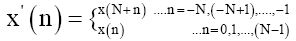The received symbol corrupted by noise and fading effects such as Rayleigh and Rician fading, it becomes

y(n) = x′(n) ∗ h(n) + ϑ(n)

Where, ϑ(n) is an AWGN sample with variance σ2 and zero mean in the nth OFDM symbol, n is the OFDM symbol index, and by Tapped- Delay line model, h(n) is impulse response as: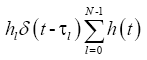Where, hl is lth gain of path channel, L is number of multipath, τl is the accompanying delay spread 0 ≤ τl ≤ τmax for most of the probable path delay τmax and each τl may be non-sample spaced. So, the discrete channel vector of L-length is as h = [h0, hl,…,h(L-1)]T

At the receiver section, putting off the CP and taking N-point DFT of the obtained vector is involved by demodulation to get

Y (k) = [Y(0), Y(1),…, Y(N-1)]T

We have every subcarrier in frequency domain.

Y(k) = X(k).H(k) + V (k)

Y = XH + V

Y = XWh + V

Where, channel frequency response (CFR) is H at subcarrier k is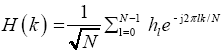While h is corresponding CIR vector and W is partial Fourier transform matrix described as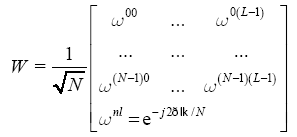Replace the matrix XW that is the approximate random Fourier transform by sensing matrix A. Similarly, in equation (1), channel impulse vector h replaces by unknown vector x and the received pilot subcarriers Y matrix by y measurement vector.

A = INR ⊗ [diag(X)W ]

Here kronecker product is represented by ⊗, we get:

y = Ax + n ... (2)

Compressive sensing is a signal processing technique for efficiently acquiring and reconstructing a signal, by finding solutions to underdetermined linear systems. In Compressive Sensing, the problem of sparse signal reconstruction is from an observed vector y ? CM to estimate a sparse x ? CN based on the linear model as equation (2). Where n ? CM is unknown noise and A? CMxN is a known measurement matrix, usually with N»M. A compressed or decreased number of measurements sensed the signal x. Therefore, problem of signal reconstruction may be explained as the following subsequent confined minimization problem,

^
x ← A† y Subject to y = Ax

Since l0 norm minimization is not computationally efficient, therefore the research focus is converted to l1 norm minimization or l2 norm minimization.

#### Modified SL0 Algorithm

Numerous wireless channels have a tendency to be over-whelmed by a moderately little number of clusters of significant paths. This inherent scarcity is exploited by the sparse channel estimation, which makes the use of compressed sensing (CS) recovery algorithms .

A thorough evidence of the convergence of the Smoothed L0 algorithm exists for a particular arrangement of parameters gave that Asymmetric Restricted Isometric Property (ARIP) limitation is fulfilled . The authors of the proof conclude that though theoretically satisfactory, the ARIP constraint promotes to an unnecessarily pessimistic choice of parameter quantities. Motivated from this conclusion, we executed a concentrated watched assessment with goal of finding the ideal parameter values. We propose an alteration to the SL0 calculation which may enormously enhance the general execution. We proposed a modified SL0 (mSL0) algorithm.

By presenting an update of L parameter, the phase transition of the SL0 algorithm can be enhanced . But computational time to get improved result is comparatively more. Subsequently to evade this downside, we keep same L parameter as that of original SL0 algorithm. By carefully selecting sequences of ús and σ’s, the phase transition of the SL0 algorithm can be further enhanced to consistently lie on or above that of the l1 approach. In brief, we chose the step-size in the order of 10-3 for the first few σ’s and in the order of 1 for the last σ’s. Also, we chose the initial σ based on information of the indeterminacy δ to improve the phase transition across all δ.

In light of our tests, we propose the accompanying method-ology. We choose a sequence of step-size of u= [1.8, 0.7, 0.4]. Furthermore, proportionally inverse relation between δ and the initial value of σ yielded the most promising phase transition. Specifically, we choose an initial σ = 1 *max|x^|.

Finally, a gradually increasing L for decreasing σ still provides an improvement for the new parameter choices. For the most part, this measure has turned out to be a good indicator of convergence and significantly reduced the average number of iterations taken in the inner loop. We now propose the modified smoothed L0 norm algorithm with enhanced parameter choice. We have proposed extraordinary change to existing calculation. Our proposed modified SL0 (mSL0) algorithm is as given below:

Modified SL0Algorithm:

Initialise:

σup = 0.5, σmin = 0.001,

u = [1.8, 0.7, 0.4],

L = 3

^
x ← A y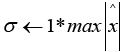for σ > σmin do

for i = 1….L do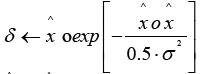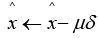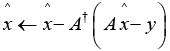σ←σ.σup

end

end

#### Simulation

We tend to indicate numerical re-enactments in simulation the planned modified SL0 (mSL0) primarily based sparse channel estimation methodology in this segment. Next, we assess SNR, BER and MSE execution. In Table 2, it is recorded that the execution of alteration of our re-enactment parameters.

Iteration MSE SNR Sigma
1 0.020493 6.489715 0.894731
2 0.005501 12.20119 0.447366
3 0.00091 20.01302 0.223683
4 0.000112 29.12282 0.111841
5 0.000016 37.50211 0.055921
6 0.000003 45.10338 0.02796
7 0.000001 49.62701 0.01398
8 0.000001 50.49605 0.00699

Table 2: Progress of Modified SL0.

BER performance

The BER execution of the BPSK-OFDM framework will profit by better MSE execution of the channel estimator. In Figure 3, the BER execution of OFDM frameworks with proposed channel estimator checks this perspective. As SNR builds, BER diminishes.

Performance in AWGN channel

Give us a chance to utilize an OFDM framework taking into account IEEE 802.11a details which are appeared in Table 3.

Parameter Value
Total Symbol Duration 4 s
Data Symbol Duration 3.2 s
Cyclic prefix duration 0.8 s
Sub-carrier spacing 312.5 kHz
FFT sampling frequency 20 MHz
FFT size 64
Number of used sub-carriers 52

Table 3: IEEE 802.11a standard.

An OFDM physical layer of the IEEE 802.11a standard decides that parts a data signal crosswise over 52 separate subcarriers. 48 subcarriers give separate wireless pathways to send the data in a parallel way and remaining 4 subcarriers are pilot or reference subcarriers. The subsequent subcarrier spacing is 0.3125 MHz (for a 20 MHz BW with 64 conceivable frequency slots). The crucial parameters for OFDM frame-works according to IEEE 802.11a standard are given in Table 3 .

In genuine, the transmission of data is much more complex than it seems in the block diagrams. Different variables impact the proficiency of the system like the density of users, line of sight between the transmitter and receiver, mutual movement between the transmitter and receiver etc. To consider these factors different channel models are developed like AWGN (Additive White Gaussian Noise) channel, Rayleigh fading channel, Rician fading channel etc.

In Figure 4, we are seeing the performance of our proposed modified SL0 (mSL0) algorithm in AWGN channel. Theoretical and estimated BER performances are shown in following Figure 4. As Eb/ N0 increases, BER goes on decreasing. Theoretical graph gives more information than estimated one.

The performance (Eb/N0 Vs BER) of BPSK modulation (with coherent detection) over Rayleigh Fading channel and its comparison over AWGN channel is discussed in this section. Here, n is the AWGN which is Gaussian distributed noise with zero mean and unit variance and A is the complex channel amplitude scaling factor that follows Rayleigh distribution.

Rayleigh fading model is utilized to simulate environments that have Non Line Of Sight (NLOS) path and numerous scatters. If there are sufficient multiple scatters in the environment, all the reflected signals that show up at the receiver end becomes uncorrelated in amplitude and phase evenly distributed between 0 to 2π (This brings mean=0).

The theoretical BER for BPSK modulation over Rayleigh fading channel with AWGN is given by: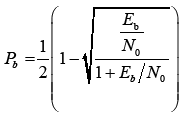The simulated and theoretical performance curves (Eb/No vs BER) for BPSK modulation over Rayleigh Fading channel and the AWGN is given in above Figure 5.

Now, let us select here channels with AWGN and Rician fading to study the behaviour of OFDM system. Rician Fading model is utilized to simulate environments that produce multipath components in addition to a dominant Line Of Sight (LOS) component. The LOS component is called specular component and the multipath components are called scatter or random components. The, the random component is considered to have zero-mean whereas, amplitude distribution of the LOS component will have non-zero mean.

In a Rician Fading environment, the non-centrality parameter (the unevenness in the means) is caused by the presence of dominant path. Because of this, the Rician K factor - representing the ratio of power of Line-Of-Sight (LOS) (or dominant multipath component) and the power of Non-Line-Of-Sight (NLOS) (or the remaining multipath components) is defined in such situation. In the following simulation model, the total power of dominant and scattered components is assumed to be unity. The results are plotted for different Rician K factors.

Let’s take up some bandwidth-efficient linear digital modulation techniques (BPSK, QPSK and QAM) and compare its performance based on their theoretical BER over AWGN.

Taking after shows theoretically that 64-QAM is the optimum modulation technique for modern wireless communication channel. We have seen that for different modern wireless communication systems, M-PSK and M-QAM are the most common modulation technique. Hence, we have chosen BPSK, 4-QAM (equivalent to QPSK), 8-QAM, 16-QAM, 32-QAM and 64-QAM to show the BER in AWGN channel. The following graph shows the BER vs Eb/No (dB) for M-QAM using AWGN channel.

In this proposed work we have exhibited a wide investigation about the significance of BER (Bit Error Rate) and Eb/No under scenario in which includes these different modulation techniques. We realize that Eb/No is expanded to a large extent with simultaneous decrease in bit error rate. We obtained the following result after doing MATLAB coding and running the program. The higher (better) the Eb/No, the better the BER. BER versus Eb/No graph is shown Figure 6.

Tables 4 and 5 summarize the theoretical BER (given SNR per bit ratio Eb/N0) for various linear modulations. Note that the Eb/N0 values used in that Table 5 are in linear scale [to convert Eb/N0 in dB to linear scale use Eb/N0 (linear) = 10(Eb/N0 (dB)/10)]. The simulation gives the following output, in Figure 7.

Modulation Detection Method Bit Error Rate
BPSK Coherent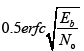QPSK Coherent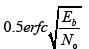M-PSK Coherent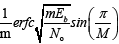M-QAM Coherent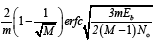Table 4: Theoretical BER over AWGN.

Modulation   Min. channel BW for no ISI
BPSR 1 Rb
QPSR 2 0.5 Rb
4-QAM 2 0.5 Rb
8-PSK 3 0.33 Rb
16-QAM 4 0.25 Rb
16-PSK 4 0.25 Rb
64-QAM 6 0.17 Rb
32-PSK 5 0.2 Rb

Table 5: Standard data of various modulation techniques for various bandwidths.

#### Conclusion

In this paper, we compare the performance in terms of BER using different fading with AWGN channel. Similarly, we evaluate Bit Error Rate. In this case we simulate different combination of modulating technique. We compared BER performance of various techniques using data transmission.

In this paper, we have evaluated the performance of BPSK with OFDM AWGN and Rayleigh fading distribution as well as Rician fading distribution was evaluated. Graphical results show the improvement in BPSK with Rayleigh fading channel compared to its performance in AWGN channel. The graphical results prove that simulated BER of BPSK is same as that of theoretical BER of BPSK. The reported BER reduced by our proposed channel estimation technique.

Graphical results show the improvement in OFDM M-PSK and M-QAM system. 64-QAM gives the comparatively good performance than 32-PSK. The results presented shows that 64-QAM performs better for both fading channels but it also increases the complexity of the system.

#### References

Citation: Mahind U, Kadam M (2016) BER Performance of OFDM System in Noise and Fading Channel for Modified SL0 Sparse Algorithm. J Telecommun Syst Manage 5: 141. Doi: 10.4172/2167-0919.1000141

Copyright: ©2016 Mahind U, et al. This is an open-access article distributed under the terms of the Creative Commons Attribution License, which permits unrestricted use, distribution, and reproduction in any medium, provided the original author and source are credited.

Select your language of interest to view the total content in your interested language

London, UK

### 6th International Conference on Wireless,Telecommunication&IoT

Barcelona, Spain
##### Recommended Journals
Viewmore
###### Article Usage
• Total views: 15343
• [From(publication date): 11-2016 - Feb 24, 2020]
• Breakdown by view type
• HTML page views: 14920Can't read the image? click here to refresh